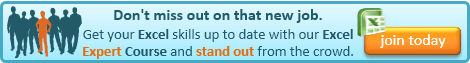From the Not Just Numbers blog:

Sometimes in your spreadsheet, like in life, it is good to get your bearings to establish where you are.

This can be very useful in a formula if you want the result to be dependent on the location in the spreadsheet.

For example, you might want to:

• format odd and even rows (or columns) differently, or
• apply a different formula depending on how far down a list an item appears, or
• populate cells with a particular value, formula or format based upon  an entered width and height.
To do any of these types of things with a formula, the formula will have to know its own location (i.e. its row and column) first.
The ROW and COLUMN commands do exactly that.
The syntax of the ROW command is:
=ROW([Reference])
where Reference is the cell reference that you wish to return the row of. So,
=ROW(A5)
for example, will return 5.
But notice that Reference is in square brackets which, you may remember form earlier posts, means that it is an optional argument. So, the function can be written as just:
=ROW()
This will return the number of the row in which the formula sits, i.e. giving us the row information that we would need for all of our examples above.
Typically we will use it in an IF statement to drive the different outcomes in our examples, for example:
=IF(ROW()<=10,1,2)
will return a 1 in rows 1 to 10 and a 2 thereafter.
The COLUMN function works exactly the same way so:
=COLUMN(A5)
returns 1 (the column is returned as a number not a letter, so A is 1, B is 2, etc. – this does make it easier for adding and subtracting column positions). Also,
=COLUMN()
returns the column number of the column in which the formula sits.
One word of caution. You need to remember that you have used these functions if you start inserting or deleting rows and columns. Most functions will adjust accordingly and maintain the same relative references, but because these refer to the actual cell positions, their results will change if their position changes.If you enjoyed this post, go to the top of the blog, where you can subscribe for regular updates and get two freebies “The 5 Excel features that you NEED to know” and “30 Chants for Better Charts”.